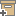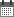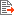ניהול
קהילה:
אסיף מאגר המחקר החקלאי
Simulation of a potential error in determining soil salinity from measured apparent electrical conductivityBack to searchPrevious item
Next item
Year:
1998
Authors :
פרידמן, שמואל
;
.
Volume :
62
Co-Authors:
Friedman, S.P., Inst. Soil, Water, and Environ. Sci., Agricultural Research Organization, Volcani Center, Bet Dagan 50250, Israel
Facilitators :
From page:
593
To page:
599
(
Total pages:
7
)
Abstract:
A common method for estimating soil salinity is by in situ measurements of the apparent electrical conductivity, EC(a), usually by a four-electrode probe. It is assumed that the EC(a) can be regarded as taking place in parallel mode by two conductors on a bulk scale: the dissolved (EC(b)) and the adsorbed (EC(s)) ions. Therefore, the contribution to the EC(a) by the soil solution electrolytes, EC(b), which serves to assess its salinity, can be deduced by subtracting the estimated EC(s) from the measured EC(a). This assumption is wrong and leads to an evaluation of EC(b) higher than its real value. This study was conducted to characterize the error in estimating soil solution electrical conductivity, EC(w). A simplified model of a randomly diluted and pore-size-distribution-decorated simple cubic lattice serves to describe the pore network of a saturated soil. It is assumed that only within each pore can the electrical conductance be represented by a sum of two conductors: the dissolved and the adsorbed ions, acting in parallel. Using Monte Carlo lattice simulations, it was shown that the error due to the assumption of parallel mode on a bulk scale increases with increasing broadness of the pore-size distribution, decreasing connectivities, and increasing cation-exchange capacity. An illustrative example of real soils, typical of irrigated soils, indicated errors of up to 25% for electrolyte concentrations.A common method for estimating soil salinity is by in situ measurements of the apparent electrical conductivity, ECa, usually by a four-electrode probe. It is assumed that the ECa can be regarded as taking place in parallel mode by two conductors on a bulk scale: the dissolved (ECb) and the adsorbed (ECs) ions. Therefore, the contribution to the ECa by the soil solution electrolytes, ECb, which serves to assess its salinity, can be deduced by subtracting the estimated ECs from the measured ECa. This assumption is wrong and leads to an evaluation of ECb higher than its real value. This study was conducted to characterize the error in estimating soil solution electrical conductivity, ECw. A simplified model of a randomly diluted and pore-size-distribution-decorated simple cubic lattice serves to describe the pore network of a saturated soil. It is assumed that only within each pore can the electrical conductance be represented by a sum of two conductors: the dissolved and the adsorbed ions, acting in parallel. Using Monte Carlo lattice simulations, it was shown that the error due to the assumption of parallel mode on a bulk scale increases with increasing broadness of the pore-size distribution, decreasing connectivities, and increasing cation-exchange capacity. An illustrative example of real soils, typical of irrigated soils, indicated errors of up to 25% for electrolyte concentrations.
Note:
Related Files :
computer simulation
Electric conductivity measurement
Error analysis
Ion exchangers
Monte Carlo methods
Soils
עוד תגיות
תוכן קשור
More details
DOI :
Article number:
Affiliations:
Database:
סקופוס
Publication Type:
מאמר
;
.
Language:
אנגלית
Editors' remarks:
ID:
31324
Last updated date:
02/03/2022 17:27
Creation date:
17/04/2018 01:01Scientific Publication
Simulation of a potential error in determining soil salinity from measured apparent electrical conductivity
62
Friedman, S.P., Inst. Soil, Water, and Environ. Sci., Agricultural Research Organization, Volcani Center, Bet Dagan 50250, Israel
Simulation of a potential error in determining soil salinity from measured apparent electrical conductivity
A common method for estimating soil salinity is by in situ measurements of the apparent electrical conductivity, EC(a), usually by a four-electrode probe. It is assumed that the EC(a) can be regarded as taking place in parallel mode by two conductors on a bulk scale: the dissolved (EC(b)) and the adsorbed (EC(s)) ions. Therefore, the contribution to the EC(a) by the soil solution electrolytes, EC(b), which serves to assess its salinity, can be deduced by subtracting the estimated EC(s) from the measured EC(a). This assumption is wrong and leads to an evaluation of EC(b) higher than its real value. This study was conducted to characterize the error in estimating soil solution electrical conductivity, EC(w). A simplified model of a randomly diluted and pore-size-distribution-decorated simple cubic lattice serves to describe the pore network of a saturated soil. It is assumed that only within each pore can the electrical conductance be represented by a sum of two conductors: the dissolved and the adsorbed ions, acting in parallel. Using Monte Carlo lattice simulations, it was shown that the error due to the assumption of parallel mode on a bulk scale increases with increasing broadness of the pore-size distribution, decreasing connectivities, and increasing cation-exchange capacity. An illustrative example of real soils, typical of irrigated soils, indicated errors of up to 25% for electrolyte concentrations.A common method for estimating soil salinity is by in situ measurements of the apparent electrical conductivity, ECa, usually by a four-electrode probe. It is assumed that the ECa can be regarded as taking place in parallel mode by two conductors on a bulk scale: the dissolved (ECb) and the adsorbed (ECs) ions. Therefore, the contribution to the ECa by the soil solution electrolytes, ECb, which serves to assess its salinity, can be deduced by subtracting the estimated ECs from the measured ECa. This assumption is wrong and leads to an evaluation of ECb higher than its real value. This study was conducted to characterize the error in estimating soil solution electrical conductivity, ECw. A simplified model of a randomly diluted and pore-size-distribution-decorated simple cubic lattice serves to describe the pore network of a saturated soil. It is assumed that only within each pore can the electrical conductance be represented by a sum of two conductors: the dissolved and the adsorbed ions, acting in parallel. Using Monte Carlo lattice simulations, it was shown that the error due to the assumption of parallel mode on a bulk scale increases with increasing broadness of the pore-size distribution, decreasing connectivities, and increasing cation-exchange capacity. An illustrative example of real soils, typical of irrigated soils, indicated errors of up to 25% for electrolyte concentrations.
Scientific Publication
You may also be interested in